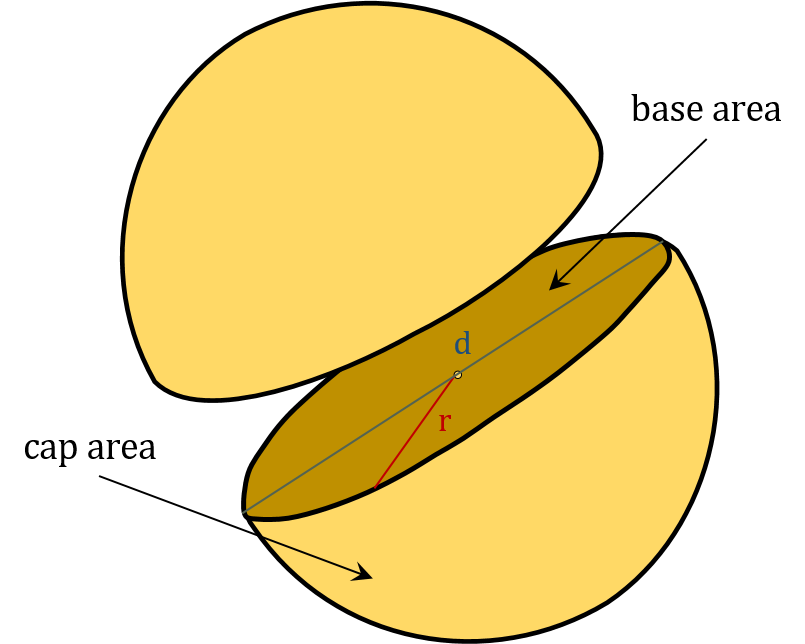# Volume of a Hemisphere Calculator

Created by Dominik Czernia, PhD candidate
Reviewed by Steven Wooding
Last updated: Feb 09, 2022

This volume of a hemisphere calculator quickly finds the volume of a hemisphere with six various equations. Do you need to solve a specific problem, but you don't know how to find the volume of a hemisphere? Try entering one of the parameters and see how simply this calculator works.

Are you curious about what principles lie behind our tool? In the following text, you will learn more about hemisphere properties and find out which of the volume of hemisphere formulas we have used. In all cases, you need only one of the below quantities:• $r$ – Radius of a hemisphere;
• $d$ – Diameter of a hemisphere;
• $V$ – Volume of a hemisphere;
• $A$ – Total surface area of a hemisphere;
• $Ab$ – Base surface area of a hemisphere;
• $Ac$ – Cap surface area of a hemisphere; and
• $A/V$ – Surface to volume ratio of a hemisphere.

As the name suggests, a hemisphere is one part of a sphere that is divided into two equal halves. The total surface area of each hemisphere consists of the base area (a circle) and cap area. On the one hand, the sum of two hemispheres surface areas is not the same as the full sphere surface area, but on the other hand, the sum of two hemispheres volumes equals the volume of a complete sphere.

## How to find the volume of a hemisphere

The simplest way to estimate the volume of a hemisphere is to imagine it as a part of a full sphere. You can find the sphere volume equation in our sphere volume calculator. It is expressed in terms of the radius:

$\quad V_{\text{sphere}} = \frac{4}{3} \pi r^3$

Therefore, the volume of a hemisphere formula is as follows:

\quad \begin{align*} V &= \frac{V_{\text{sphere}}}{2}\\\\ V &= \frac{2}{3} \pi r^3 \end{align*}

The situation is a little more complicated with the surface area. If you want to determine the differences between sphere and hemisphere areas, head to the area of a hemisphere calculator. Briefly speaking, the hemisphere has an additional base area, which you should take into account.

With our volume of a hemisphere calculator, you can perform calculations in many different units (including SI and imperial units). Don't hesitate and check out our area and volume converters to learn more about various unit conversions!

## What's the volume of a hemisphere formula?

How to find the volume of a hemisphere when we don't have a radius given? It depends on what you know about a specific hemisphere. Our volume of a hemisphere calculator can estimate, from one chosen parameter, all the other hemisphere quantities. To explain that, let's start by listing some basic equations that describe the properties of a hemisphere:

1. Diameter of a hemisphere: $d = 2r$,
2. Volume of a hemisphere: $V = \frac{2}{3} \pi r^3$,
3. Base surface area of a hemisphere: $Ab = \pi r^2$,
4. Cap surface area of a hemisphere: $Ac = 2 \pi r^2$,
5. Total surface area of a hemisphere: $A = 3 \pi r^2$,
6. Surface to volume ratio of a hemisphere: $A / V = 9 / 2 r$.

After some simple algebraic transformations, with the above equations, we can finally write six explicit volume of a hemisphere formulas that are used by our volume of a hemisphere calculator:

1. Given radius: $V = \frac{2}{3} \pi r^3$,
2. Given diameter: $V = \frac{1}{12} \pi d^3$,
3. Given base area: $V = \frac{2}{3} \sqrt{Ab^3 / \pi}$,
4. Given cap area: $V = \frac{1}{3} \sqrt{Ac^3 / 2 π}$,
5. Given total area: $V = \frac{2}{9} \sqrt{A³ / 3 \pi}$,
6. Given surface to volume ratio: $V = 243 \pi / 4 (A/V)^3$.

You can find hemispheres in many aspects of our life. Many of the surrounding objects are shaped like half of a sphere. In geography and physics, we often say that the Earth is divided into hemispheres: northern and southern. Did you know that when you travel by airplane, "an invisible" force acts on you, taking you off the course? That effect is called the Coriolis effect, and it behaves differently on the northern and southern hemispheres of the Earth.

## FAQ

### What is half a sphere called?

A hemisphere. This word comes from the Greek word "hemi", meaning "half", and the Latin word "shaera", meaning "globe". That's why we divided the Earth into hemispheres, north and south and also east and west.

### How do I calculate the surface area of a hemisphere?

To calculate the surface area of a hemisphere:

1. Square the radius of the hemisphere.
2. Multiply by the number pi.
3. Multiply by three.

Mathematically, that's A = 3𝜋r².

### How many faces are on a hemisphere?

A hemisphere has one face, which is the flat base. The dome part is classified as a curved surface. A hemisphere also has one circle edge and no vertices.

### How do I calculate the volume of a hemisphere given its diameter?

If you know the diameter of a hemisphere, here is how to calculator its volume:

1. Cube the diameter.
2. Multiply by the number pi.
3. Divide by the number 12.
Dominik Czernia, PhD candidatein
Diameter
in
Surface to volume ratio
1/
in
Surface areas
Base
in²
Cap
in²
Total
in²
Volume
Volume
cu in
People also viewed…

### Eggs

Quantum physicist's take on boiling a perfect egg.

### Parallelogram Perimeter

Parallelogram perimeter calculator finds out the perimeter of a parallelogram applying three useful formulas.

### Plastic Footprint

Find out how much plastic you use throughout a year with this plastic footprint calculator. Rethink your habits, reduce your plastic waste, and make your life a little greener.

### Rectangle scale factor

Calculate linear and area scale factors between two rectangles using this rectangle scale factor calculator.